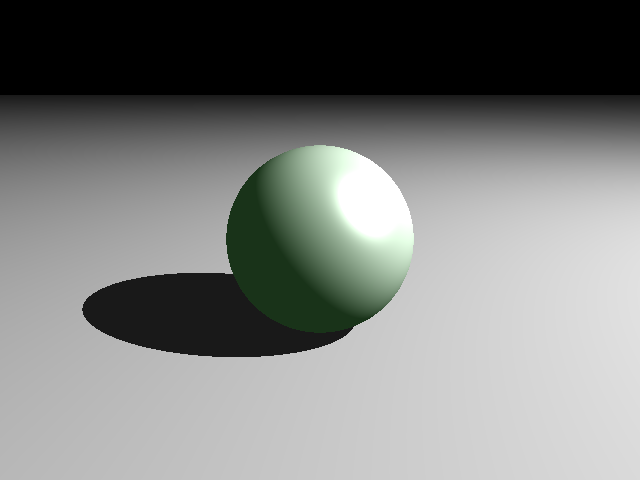``````cargo new raytracing-rust –bin
``````

``````[dependencies]
time = "0.1"
``````

``````extern crate time;
use time::precise_time_ns;

let t1 = precise_time_ns;
let t2 = precise_time_ns;
``````

``````[dependencies]
bmp = "0.1.4"
``````
``````extern crate bmp;
use bmp::{Image, Pixel};
``````

``````let fx = x as f64;
let fy = y as f64;
if ASPECT > 1.0 {
xamnt = ((fx+0.5)/FWIDTH)*ASPECT - ((FWIDTH-FHEIGHT) / FHEIGHT / 2f64);
yamnt = ((FHEIGHT - fy) + 0.5)/FHEIGHT;
} else if ASPECT < 1.0 {
xamnt = (fx+0.5)/FWIDTH;
yamnt = (((FHEIGHT - fy)+0.5)/ FHEIGHT)/ASPECT - ((FHEIGHT-FWIDTH)/ FWIDTH/2.0);
} else {
xamnt = (fx+0.5)/FWIDTH;
yamnt = ((FHEIGHT - fy) + 0.5)/FHEIGHT;
}
``````

FWIDTH跟FHEIGHT都是已經轉成f64 的width, height，Rust 在四則運算上，就限制了float 不能跟int, unsigned int 之類的運算， 你一定要自己轉型不然 rustc 就跟你該該叫。

Rust 也能用一些相當高階的寫法，很多C/C++裡的index based for loop 在這裡都可以簡化 （當然這樣寫是不是比較快？有沒有必要這樣寫倒不一定），例如要求某個陣列中的最大值，如果是for loop 寫法會是這樣：

``````let mut max = 0.0f64;
for intersect in intersections.iter() {
if *intersect > max {
max = *intersect
}
}
``````

``````let mut max = intersections.iter().fold(0.0f64, |max, &x| x.max(max));
``````

``````let mut second_intersect :Vec = Vec::new();
for obj in scene_obj.iter() {
}
for d in second_intersect {
if d > ACCURACY && d <= light_dis {
// object between light and intersect point
break;
}
}
``````

``````let shadowed = scene_obj
.iter()
.any(|x| x>ACCURACY && x< light_dis);
``````## 後記#

• 寫這個就會覺得我當初3DMM 沒學好，對不起簡教授QAQ
• 算是小小吐槽一下好了，原本的那部影片用的根本是用了 Class 跟 Vector 的 C
我邊看邊Murmur: 你這樣寫你到底會不會寫C++ 呀幹。
然後有些地方的寫法，例如它Sphere 的findIntersection()，
把各個vector 翻出來開腸剖肚的寫法，你真的有想過這些東西都是向量運算，之前你就實作過了，你寫了 20 行的東西我兩行就寫完了耶XD